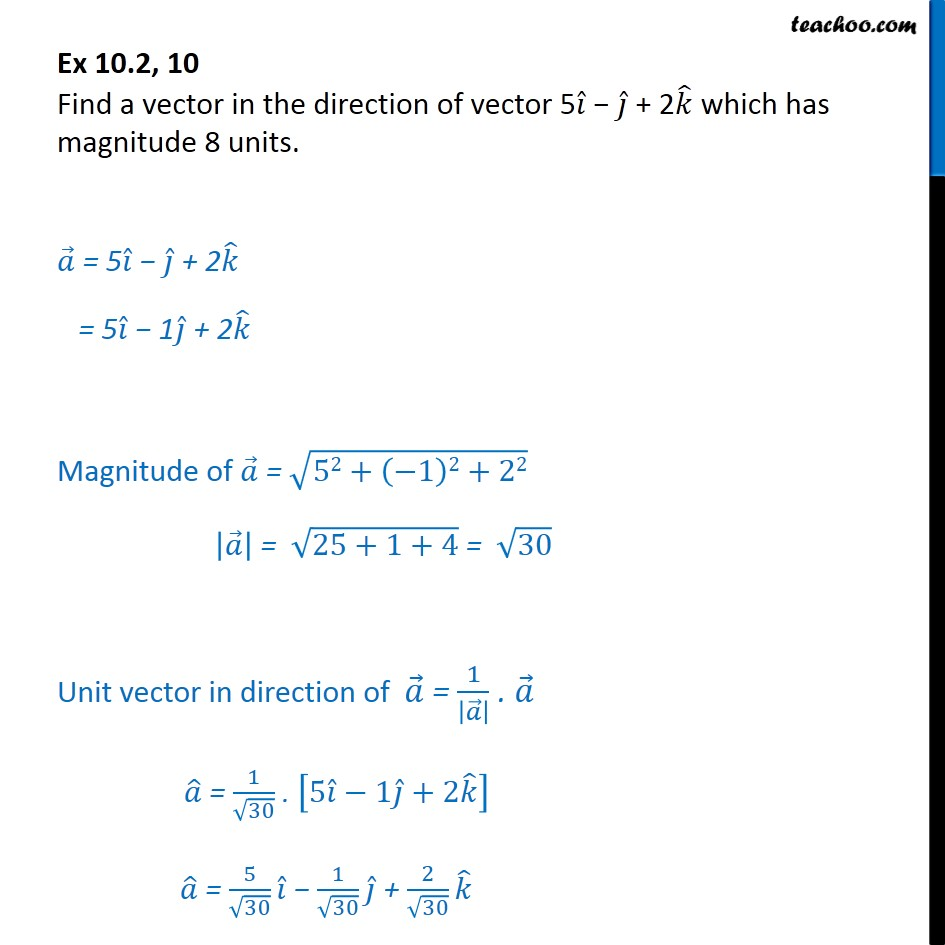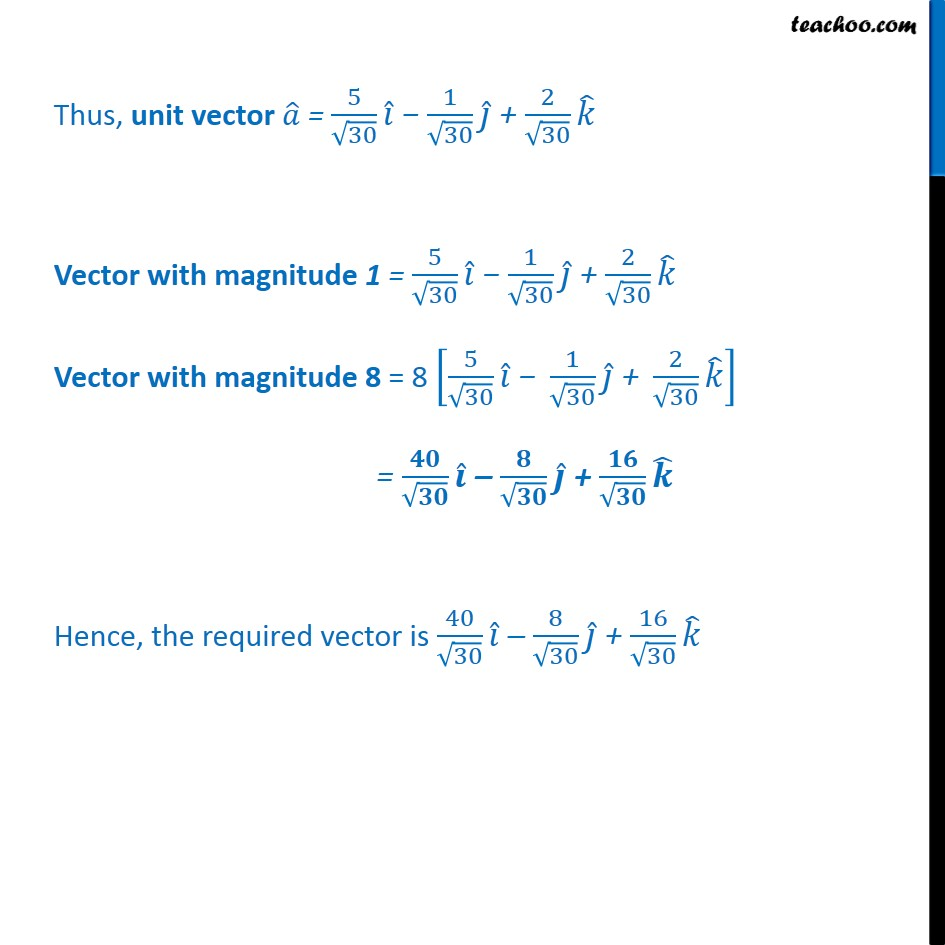1. Class 12
2. Important Question for exams Class 12
3. Chapter 10 Class 12 Vector Algebra

Transcript

Ex 10.2, 10 Find a vector in the direction of vector 5 + 2 which has magnitude 8 units. = 5 + 2 = 5 1 + 2 Magnitude of = 52+ 1 2+22 = 25+1+4 = 30 Unit vector in direction of = 1 . = 1 30 . 5 1 +2 = 5 30 1 30 + 2 30 Thus, unit vector = 5 30 1 30 + 2 30 Vector with magnitude 1 = 5 30 1 30 + 2 30 Vector with magnitude 8 = 8 5 30 1 30 + 2 30 = + Hence, the required vector is 40 30 8 30 + 16 30

Chapter 10 Class 12 Vector Algebra

Class 12
Important Question for exams Class 12